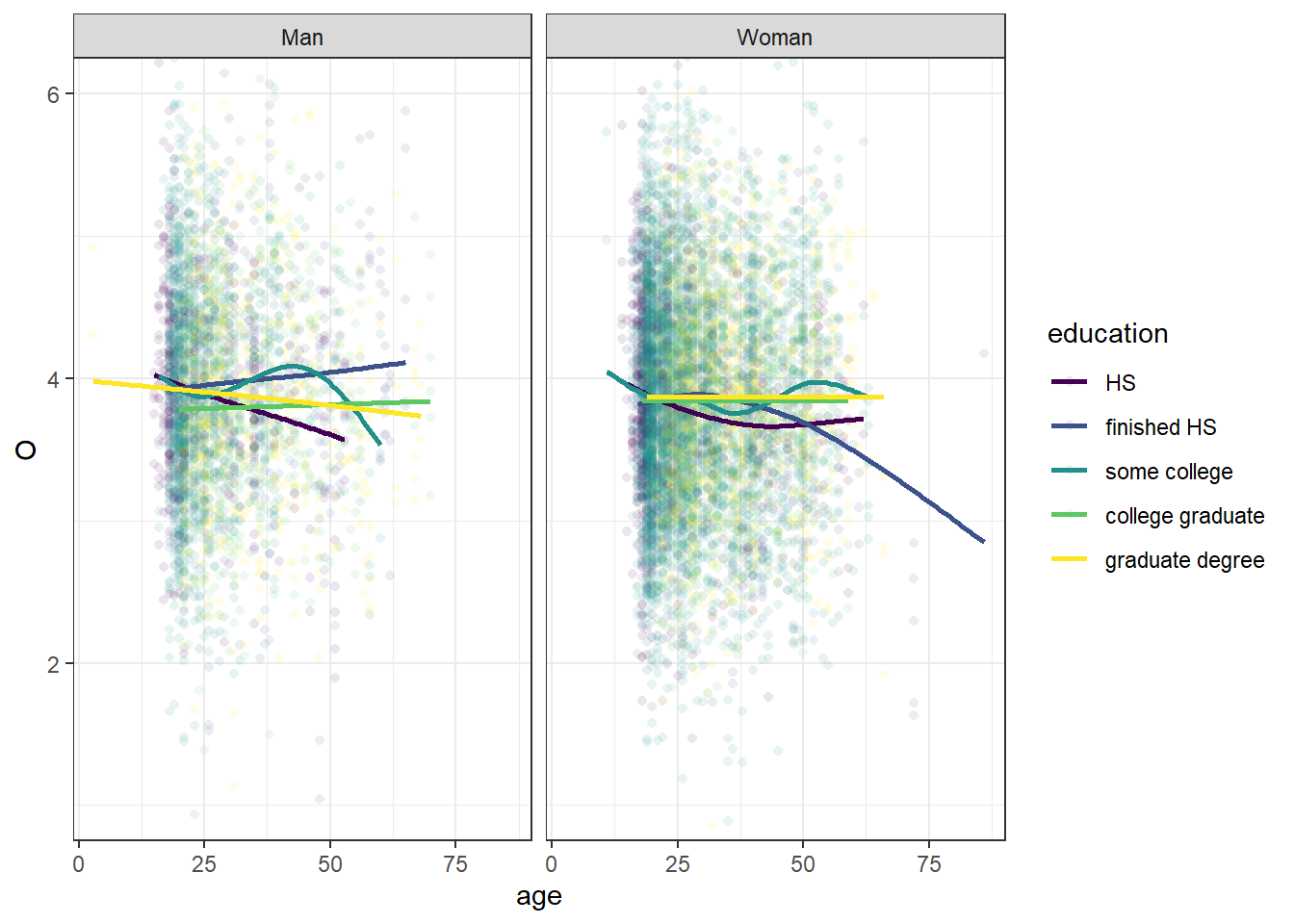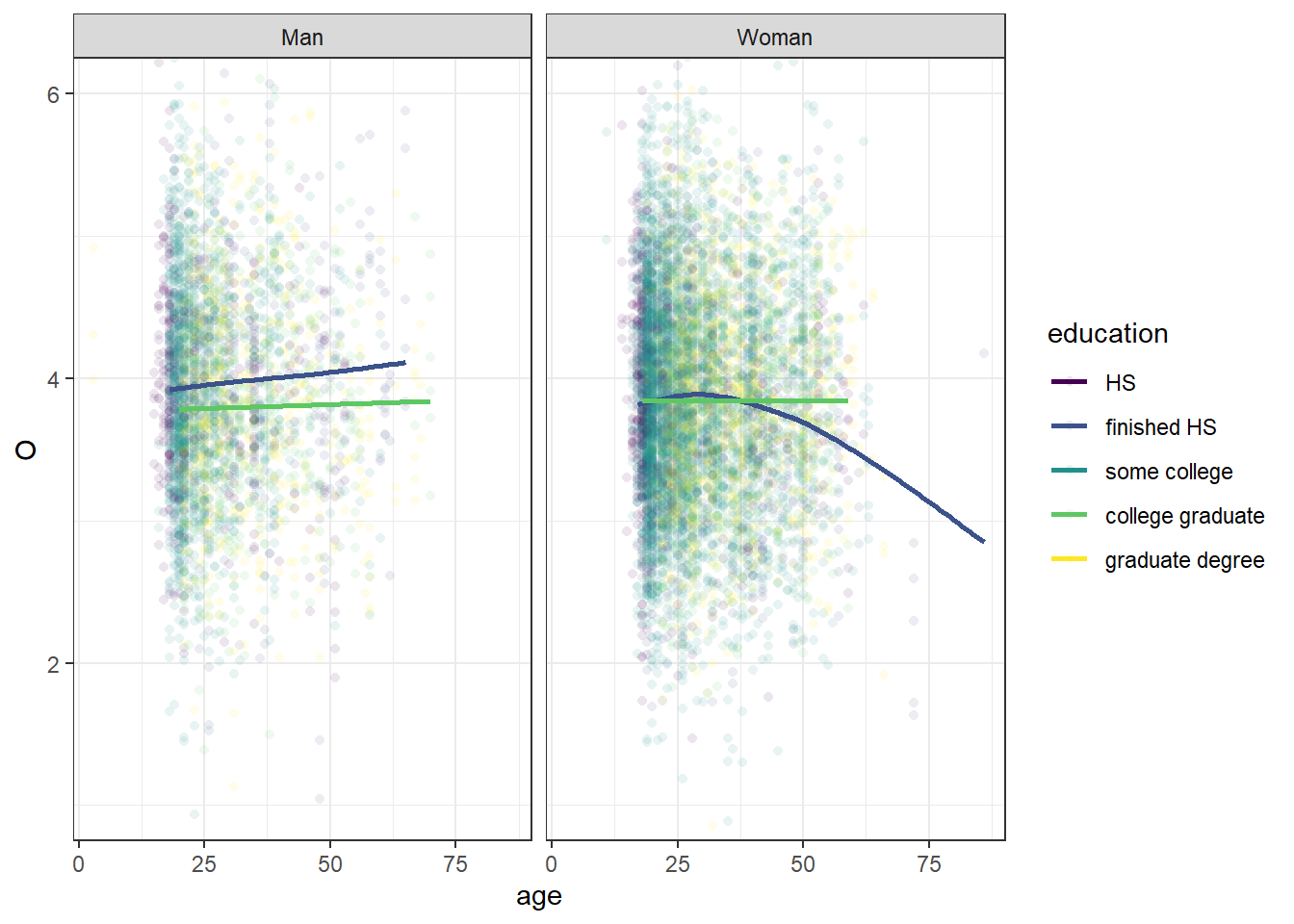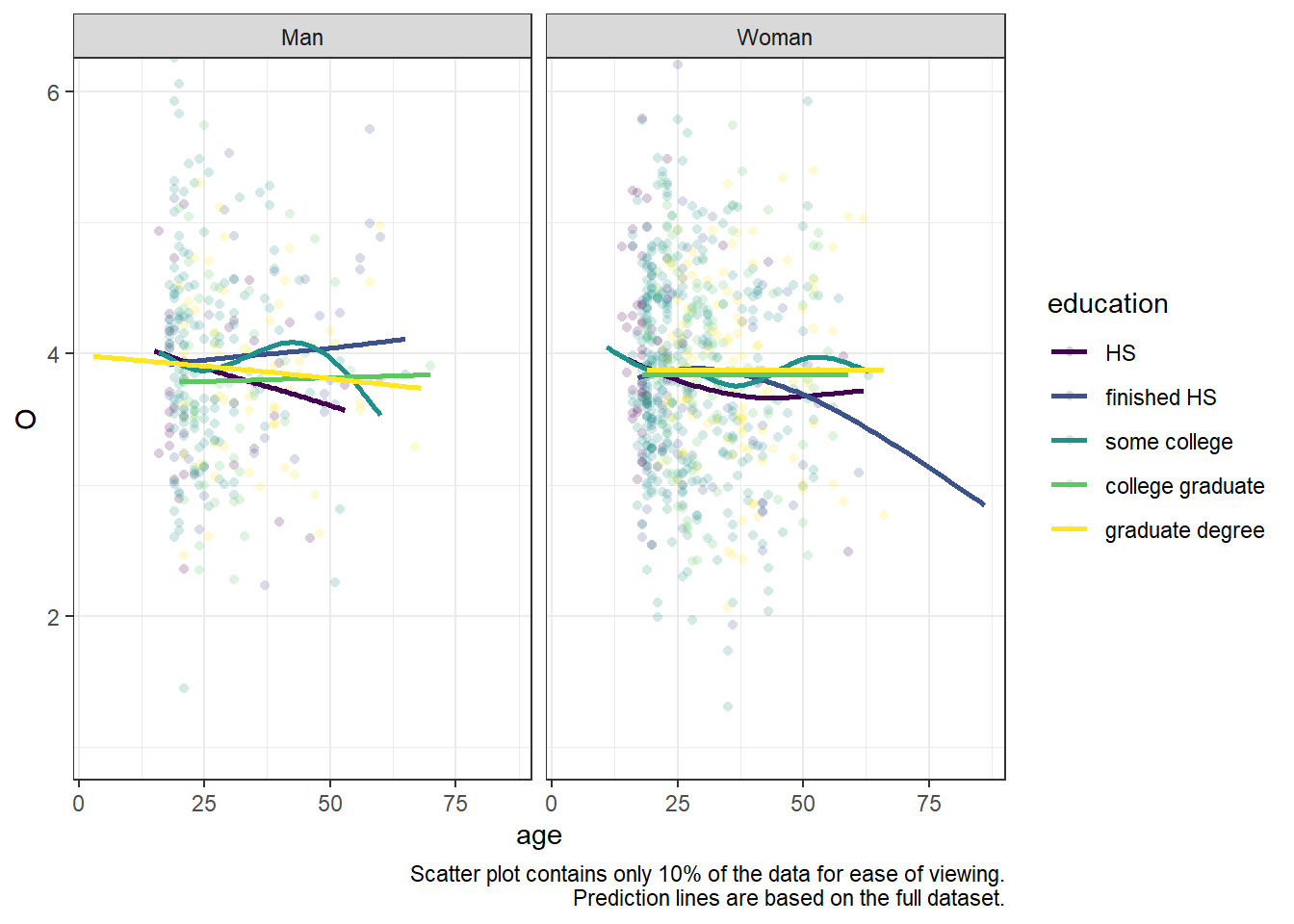# ggplot: plot only some of the data

R
ggplot2
Author

Mattan S. Ben-Shachar

Published

July 12, 2021

Often (especially when working with large and/or rich datasets) our (gg)plots can feel cluttered with information. But they don’t have to be!

Let’s look at the following plot:

Generate some data
``````library(dplyr)

bfi <- psychTools::bfi %>%
mutate(
O = across(starts_with("O")) %>% rowMeans(na.rm = TRUE),
C = across(starts_with("C")) %>% rowMeans(na.rm = TRUE),
E = across(starts_with("E")) %>% rowMeans(na.rm = TRUE),
A = across(starts_with("A")) %>% rowMeans(na.rm = TRUE),
N = across(starts_with("N")) %>% rowMeans(na.rm = TRUE)
) %>%
mutate(
gender = factor(gender, labels = c("Man", "Woman")),
education = factor(education, labels = c("HS", "finished HS", "some college", "college graduate", "graduate degree"))
) %>%
select(gender, education, age, O:N) %>%
tidyr::drop_na(education) %>%
# multiply the data set
sample_n(size = 10000, replace = TRUE) %>%
mutate(across(O:N, \(x) x + rnorm(x, 0, sd(x))))``````
``````library(ggplot2)

theme_set(theme_bw())

base_plot <- ggplot(bfi, aes(age, O, color = education)) +
facet_wrap(facets = vars(gender)) +
coord_cartesian(ylim = c(1, 6)) +
scale_color_viridis_d()

base_plot +
geom_point(shape = 16, alpha = 0.1) +
geom_smooth(se = FALSE)``````This is a busy plot. It’s hard to see what the each prediction line is doing because there are so many of them, and it’s hard to make out the scatter plot behind the lines due to there being so many dots.

We might be tempted to pre-process some data in some way and pass it to each layer via the `data=` argument in `geom_point/smooth`. However, that gets ugly fast (did I clean the outliers in all the datasets for this plot?).

Instead, we can take advantage of the fact that the `data=` argument can take a function to pre-process that plot data before plotting:

A `function` will be called with a single argument, the plot data. The return value must be a `data.frame`, and will be used as the layer data. A `function` can be created from a formula (e.g. `~ head(.x, 10)`).

Let’s look at two examples.

## 1. Only Some of the Smooths

For example, we can pass a filtering function (here as a formula) to only get the prediction lines of two categories.

``````library(dplyr)

base_plot +
geom_point(shape = 16, alpha = 0.1) +
geom_smooth(
se = FALSE,
# looking only at HS vs collage graduates
data = ~ filter(.x, education %in% c("finished HS", "college graduate"))
)``````## 2. Only Some of the Points

We can also randomly select some points (note that the prediction lines are still based on the full dataset).

``````base_plot +
geom_point(
shape = 16, alpha = 0.2,
# sample 10% from each group of `education`+`gender`
data = ~ group_by(.x, education, gender) %>% sample_frac(0.1)
) +
geom_smooth(se = FALSE) +
# Let the reader know what you've done
labs(caption = "Scatter plot contains only 10% of the data for ease of viewing.
Prediction lines are based on the full dataset.")``````You might also be interested in `gghighlight` for plotting all of the data, but highlighting only some of it.